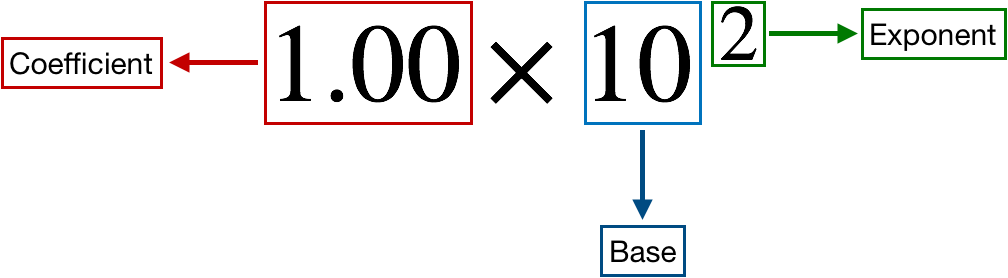# Problem: Express the following quantities in scientific notation using fundamental SI units of mass and length given in the table below.The Seven Fundamental SI Units of MeasurePhysical QuantityName of UnitAbbreviationMasskilogramkgLengthmetermTemperaturekelvinKAmount of substancemolemolTimesecondsElectric currentampereALuminous intensitycandelacdThe mass of carbon dioxide emitted from a large power plant each year, 20 Tg.

###### FREE Expert Solution

We are asked to express the mass of carbon dioxide, 20 Tg (teragram),  in scientific notation using fundamental SI units of mass and length

Recall:

1 Tg = 1012 g

scientific notation expression is composed of three parts:91% (36 ratings)###### Problem Details
Express the following quantities in scientific notation using fundamental SI units of mass and length given in the table below.

 The Seven Fundamental SI Units of Measure Physical Quantity Name of Unit Abbreviation Mass kilogram kg Length meter m Temperature kelvin K Amount of substance mole mol Time second s Electric current ampere A Luminous intensity candela cd

The mass of carbon dioxide emitted from a large power plant each year, 20 Tg.